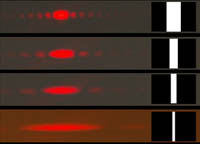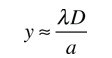# Single Slit Diffraction

## Variation with wavelength and slit widthLight which passes through a narrow slit does not produce a sharp, geometrical shadow which images the slit, but a distributed pattern of light intensity produced by diffraction. These diagrams are conceptual only - they are approximately scaled with visible color and slit width.Illustration of variation with slit width
Index

Diffraction concepts

Fraunhofer diffraction

Fraunhofer intensity concepts

 HyperPhysics***** Light and Vision R Nave
Go Back

# Single Slit Approximations

The usual approach to single slit diffraction uses the small angle approximation. There are circumstances in which this approximation needs to be examined.Examining the first minimum from the single slit givesSolving this for y gives:compared to the usual approximation :For a slit of width a = micrometers = x10^ m

 and light wavelength λ = nm for first order, m=1

on a screen at distance D = cm

the direct geometrical solution for the first minimum of intensity givescm.

 The usual approximation gives= cm

For the parameters given, the deviation is %.

Index

Diffraction concepts

Fraunhofer diffraction

Fraunhofer intensity concepts

 HyperPhysics***** Light and Vision R Nave
Go Back